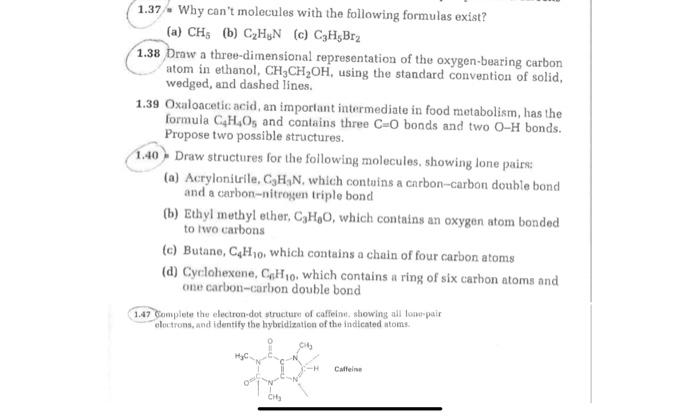Home / Expert Answers / Chemistry / could-you-possibly-help-me-on-the-other-ones-that-are-circled-nbsp-1-37-why-can-39-t-molecules-with-pa960

# (Solved): could you possibly help me on the other ones that are circled?  1.37 / Why can't molecules with ...

could you possibly help me on the other ones that are circled?1.37 / Why can't molecules with the following formulas exist? (a) $$\mathrm{CH}_{5}$$ (b) $$\mathrm{C}_{2} \mathrm{H}_{8} \mathrm{~N}$$ (c) $$\mathrm{C}_{3} \mathrm{H}_{5} \mathrm{Br}_{2}$$ $$1.38$$ Draw a three-dimensional representation of the oxygen-bearing carbon atom in ethanol, $$\mathrm{CH}_{3} \mathrm{CH}_{2} \mathrm{OH}$$, using the standard convention of solid, wedged, and dashed lines. 1.39 Oxaloacetic acid, an important intermediate in food metabolism, has the formula $$\mathrm{C}_{4} \mathrm{H}_{4} \mathrm{O}_{5}$$ and contains three $$\mathrm{C}=\mathrm{O}$$ bonds and two $$\mathrm{O}-\mathrm{H}$$ bonds. Propose two possible structures. 1.40 . Draw structures for the following molecules, showing lone pairs: (a) Acrylonitrile, $$\mathrm{C}_{3} \mathrm{H}_{3} \mathrm{~N}$$, which contuins a carbon-carbon double bond and a carbon-nitrosen triple bond (b) Ethyl methyl ether, $$\mathrm{C}_{3} \mathrm{H}_{0} \mathrm{O}$$, which contains an oxygen atom bonded to two carbons (c) Butane, $$\mathrm{C}_{4} \mathrm{H}_{10}$$, which contains a chain of four carbon atoms (d) Cyclohexene, $$\mathrm{C}_{n} \mathrm{H}_{10}$$, which contains a ring of six carhon atoms and one carbon-carbon double bond 1.47 Conesplete the electron-dot structure of caffeinn, showing all lonerpair eloctrons, and identify the hybridization of the indicated atoms.

We have an Answer from Expert